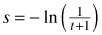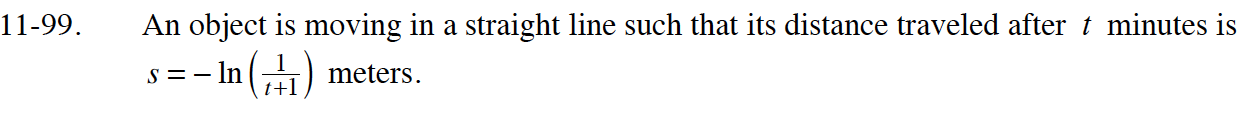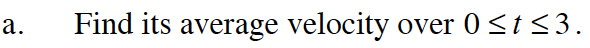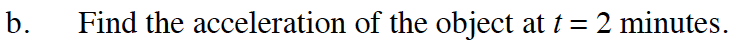### Home > CALC > Chapter 11 > Lesson 11.3.1 > Problem11-99

11-99.
1. An object is moving in a straight line such that its distance traveled after t minutes ismeters. Homework Help ✎

1. Find its average velocity over 0 ≤ t ≤ 3.

2. Find the acceleration of the object at t = 2 minutes.First, graph the equation and make sure the object does not change directions.
average velocity = (total distance)/(total time)

total distance = s(3) – s(0)s(t) = ln(t + 1)